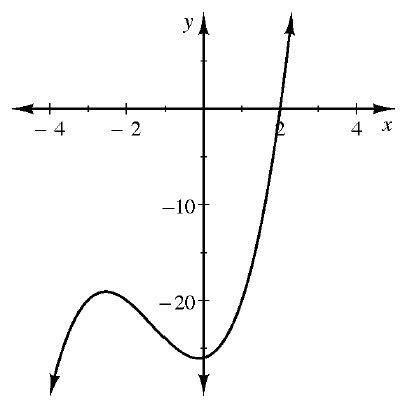### Home > INT3 > Chapter 8 > Lesson 8.3.2 > Problem8-113

8-113.

The graph of $p\left(x\right) = x^{3} + 4x^{2} + x – 26$ is shown at right.

1. Use the graph to determine one solution to $p\left(x\right) = 0$, then use the Factor Theorem to write a factor of $p\left(x\right)$. Read about the Factor Theorem in the Math Notes box in this lesson.

Where does the graph cross the $x$-axis? What is the associated factor?

2. Determine all the factors and roots of $p\left(x\right)$, including any with complex coefficients.

Divide the factor from part (a) into $p\left(x\right)$ to obtain a quadratic factor.
Then use the Quadratic Formula to find the roots.
Use the roots write the associated factors.